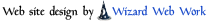Devices << VirtualDevices << WaveformTriangleDevice Software Overview  |  Sitemap  |  Downloads  |  Developers  |  ForumsHome Devices FAQs How-Tos Projects Tutorials Recipes

# Triangle Waveform DeviceThe Triangle Waveform Device generates output values in the shape of a triangle - when its output values are graphed versus time, they look like triangles. As an example, a triangle output which goes between 0 and 2 might output the following values: [0, 1, 2, 1, 0, 1, 2, 1, ....]. These values would repeat forever, with each value equidistant in time from the next.

Triangle Devices begin by outputting their minimum value, ramp up to their maximum, and then ramp down to their minimum. The pattern is repeated over and over, until the Virtual Wiring system is shutdown or restarted.

To fully define a triangle, we use the following parameters: maximum height, minimum height, step size, and delay. The minimum and maximum heights define the minimum and maximum output values (in our example, these values were 0 and 2). Step size is the number of units between one output value and the next (in our example, this value was 1). The delay is the time delay between successive output values.

## Creating a Triangle Waveform Device

Triangle Waveform Devices are created by running the WaveformTriangle Script. The Script is in the Scripts/Device/Virtual directory. The Triangle Waveform Device has one output terminal, "out", which generates the waveform. The WaveformTriangle Script takes 4 parameters: "min", "max", "step" and "delay".

• `min : the lowest possible value of the output.`

• `max : the highest possible value of the output.`

• `step : the difference between two successive output values.`

• `delay : the delay, in seconds, between 2 successive output values.`

If a step value will cause the output value to exceed its "max" or "min" value, the output will be held within the min/max range. So if one created a Triangle Device with a min of 2 and a max of 6 and a step size of 3, the output would be [2, 5, 6, 3, 2, 5, ...]. Even though the step size will send the output to 8, the max parameter forces the value to be within range(6). On the way down, the min parameter forces the output to 2, rather than letting it go to 0.

Running the WaveformTriangle Script with the parameters listed below will create a triangle waveform which starts at -1 and ramps up to 9, 2 steps at a time, each step 3 seconds apart:

`id: "my_triangle"`
`min: -1`
`max: 9`
`step: 2`
`delay: 3`

## Catalina Computing, LLC.